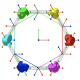# Rotate a cube to make it stand on one of its corners

Kind of a theorical question here. I would like to know how you would rotate a cube so it stands on one of its tops. I finally managed to do it but probably not in a proper way, and as i'm curious to improve my workflow, I ask you.

Maybe there is a "mathematical" way to compute the Y angle; or even better, would there be a "blender" way to do it even more quickly ? (with snapping or something)

Thanks !Somewhat better non-mathematical solution:

1. Extrude one of the side edges along the 'Z axis' and split the extrusion with Y.
2. Set cursor location with Shift+S and pivot point with comma.
3. Enable vertex snapping, set 'Edge' snap element and 'Active' snap target.
4. Select the main object with L and make one vertex active with Shift-Click.
5. Rotate in front view and side view.

That's how it looks:• While all answers here are really good, I think this is the most efficient way for me. Thanks ! – gordie Nov 11 '15 at 1:38
• But the problem with this nice technique is that you work in edit mode, and thus the rotation isn't applied on the object but on the mesh... – gordie Nov 16 '15 at 18:41

Here is a mathematical solution. When you have finished your first rotation you have something like this (a cube staying on its edge):I added a diagonal for this explanation. From the side view it looks like this:When the cube stays on its edge, the new object height is. The angle for rotation is defined by formula. To perform this rotation type in 3D view: ry=atan(1/sqrt(2))*180/pi. This will give you a perfectly oriented cube.

• And if you don't need perfect mathematical precision, just need to do it faster than eyeball, ry=35.26. – Kroltan Nov 11 '15 at 14:20

Late to the party so adding an "out of the box" fun solution, use physics. (Not to be taken seriously really but it could work if you increase the simulation steps, wait for the entire sim to end and then convert to a mesh from the baked simulation, remove the "string" and set an origin on the cube).

Example below is terrible as well since I use cloth as opposed to rigid body, the point is made however.Can't speak for mathematical ways, but you can do it like this.

1. create a "segment object" which connects your object "poles" (nort/south)
2. parent the cube to the "segment object"
3. create a vertical "reference segment", along Z
4. using snapping to edges, rotate the "segment object" from front/side views, snapping it to the "reference segment"

likeyou'll get thisMathematically

if you look at the Front Ortho of the image there is the triangle ABC. Length B is the side of the cube L, length A is the length of a faces diagonal L * sqrt(2) (basic pythagoras). The tangent of angle alpha will be L / (L * sqrt(2)) or 1 / sqrt(2) atan of which equates to 35.2644 degrees.

The rotation_euler XYZ (45, 35.264, 0)

The length of the longest diagonal C is L * sqrt(3), Once again from pythagoras. If the origin is in the middle of the cube translate the cube L * sqrt(3) / 2 to put it on its point.

Interestinglythe volume can be calculated using C**3 / (3*sqrt(3))Here's my solution outside of Blender, and I'm working to get it inside Blender. OK, only six edges and six corners of the cube remain, but that's a good start. And the two missing corners lie on the vertical blue axis in the center of the image. On a more serious note, R Z 45, R X 180*acos(sqrt(3)/3)/pi, G Z sqrt(3) to move tip to origin. Remove the two vertices on the z-axis and get the shape in the image below.Fast---

In object mode ortho 1, rotate cube by eye so vetex is in line with axis. Zoom in if you want to be accurate.

Go to ortho 3 and repeat.

Good enough for me.

... or have I missed something?

• That's what I did myself before asking this question. Good enough, but not perfect :) Anyway, thanks you all for your nice answers ! – gordie Nov 11 '15 at 1:36
• My foray into level design taught me that being exact rarely matters, and eye-balling things gives a softer, more human touch. There are legitimate cases when you'll need to be exact, but one should know with discretion whether and when they actually need exact, and when they do not. – ThorSummoner Nov 17 '15 at 10:00